## Three Coupled Cells with Brusselator Reaction

The trimolecular reaction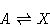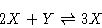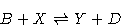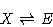was introduced in P. Glansdorff, I. Prigogine: Thermodynamic Theory of Structure, Stability and Fluctuations. Wiley-Interscience, London 1971. The concentrations of the chemicals A, B, D, E are assumed to remain at a constant level. Taking all direct kinetic constants equal to one, and neglecting the reverse processes, the reaction is described by the differential equations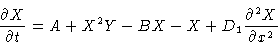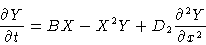The variable x measures the length of the reactor. In what follows, we shall concentrate on the kinetic equations--that is, we neglect the diffusion terms (D1 =D2 =0). Here we consider the steady-state situation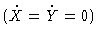in three connected reaction cells with coefficients A=2, B=6. y1 and y2 stand for X and Y in the first cell, and so on.

An inspection of the equations reveals that an exchange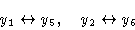yields the same equations. After a permutation of the order of equations, we arrive back at the original equation. This process of exchanging is described by the transformation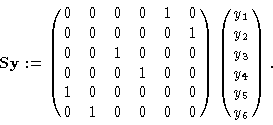We realize that this transformation and the equation written as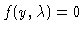satisfy the equivariance condition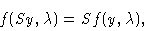and S2=I holds. That is, the equation is Z2 symmetric. Hence, in this example, we expect pitchfork bifurcations with symmetry breaking.

As an inspection of the equations shows, there is a stationary solution for all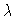,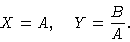For our choice of parameters this solution is given by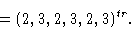We restrict a numerical analysis to the parameter range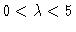. The resulting branching diagram is depicted in Figure 1, and a detail for small values ofis shown in Figure 2. As these figures show, there are a great number of turning points, pitchfork bifurcations, and transcritical bifurcations. The branch points are listed in the Table (rounded to six digits). In addition, Hopf bifurcations exist.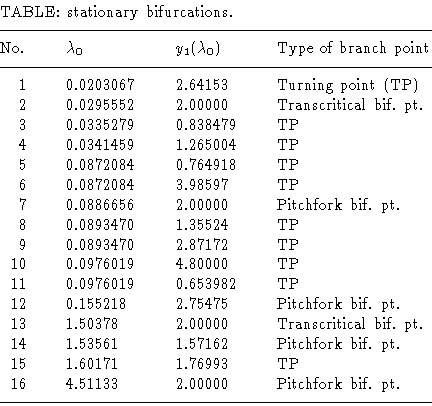Figure 3 condenses the bifurcation behavior in a qualitative way. The nontrivial branches are connected with the trivial branch via bifurcation points 2, 7, 13, 16. Because only two are pitchfork bifurcations, the way symmetries are broken or preserved is especially interesting. The trivial branch reflects a higher" regularity than the symmetry condition with S because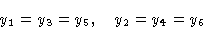holds. In Figure 3, symmetric solutions with respect to S are indicated by solid lines, and branches of asymmetric solutions are dashed, or dotted. The symmetry defined by the matrix S is preserved at the transcritical bifurcation points 2 and 13, but at these points the regularity responsible for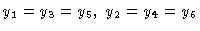is broken. Symmetry breaking of the Z2 symmetry takes place at the pitchfork bifurcations 7, 12, 14, 16.

Figure 1
Bifurcation diagram y1 versus.

Figure 2
y1 versus, detail of Figure 1.

Figure 3
Schematic bifurcation; for numbers of bifurcations see Table.

This Example
Figure 1
Figure 2
Figure 3